# How to Calculate and Solve for the Length of a Side and Area of a Hexagon | The Calculator Encyclopedia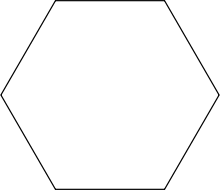The image above is a hexagon.

To compute the area of a hexagon, one essential parameter is needed and this parameter is length of side (a).

The formula for calculating the area of a hexagon:

A = (a2)3√32

Where;
A = Area of the hexagon
a = Length of side

Let’s solve an example;
Find the area of a hexagon when the length of side is 35 cm.

A = (a2)3√32
A = (352)3√32
A = 1225 x 3(1.73)2
A = 1225 x 5.1962
A = 6365.2872
A = 3182.64

Therefore, the area of the hexagon is 3182.64 cm2.

Calculating the length of side (a) using the area of the hexagon.

a = √(2A3√3)

Where;
a = length of side
A = Area of the hexagon

Let’s solve an example;
Given that the area of the hexagon is 120 cm2. Find the length of side?

This implies that;
A = Area of the hexagon = 120 cm2

a = √(2A3√3)
a = √(2 x 1205.196)
a = √(2405.196)
a = √46.189
a = 6.796

Therefore, the length of side (a) is 6.796 cm.

Nickzom Calculator – The Calculator Encyclopedia is capable of calculating the area of a hexagon.

To get the answer and workings of the area of a hexagon using the Nickzom Calculator – The Calculator Encyclopedia. First, you need to obtain the app.

You can get this app via any of these means:

To get access to the professional version via web, you need to register and subscribe for NGN 1,500 per annum to have utter access to all functionalities.
You can also try the demo version via https://www.nickzom.org/calculator

Once, you have obtained the calculator encyclopedia app, proceed to the Calculator Map, then click on Mensuration under the Mathematics section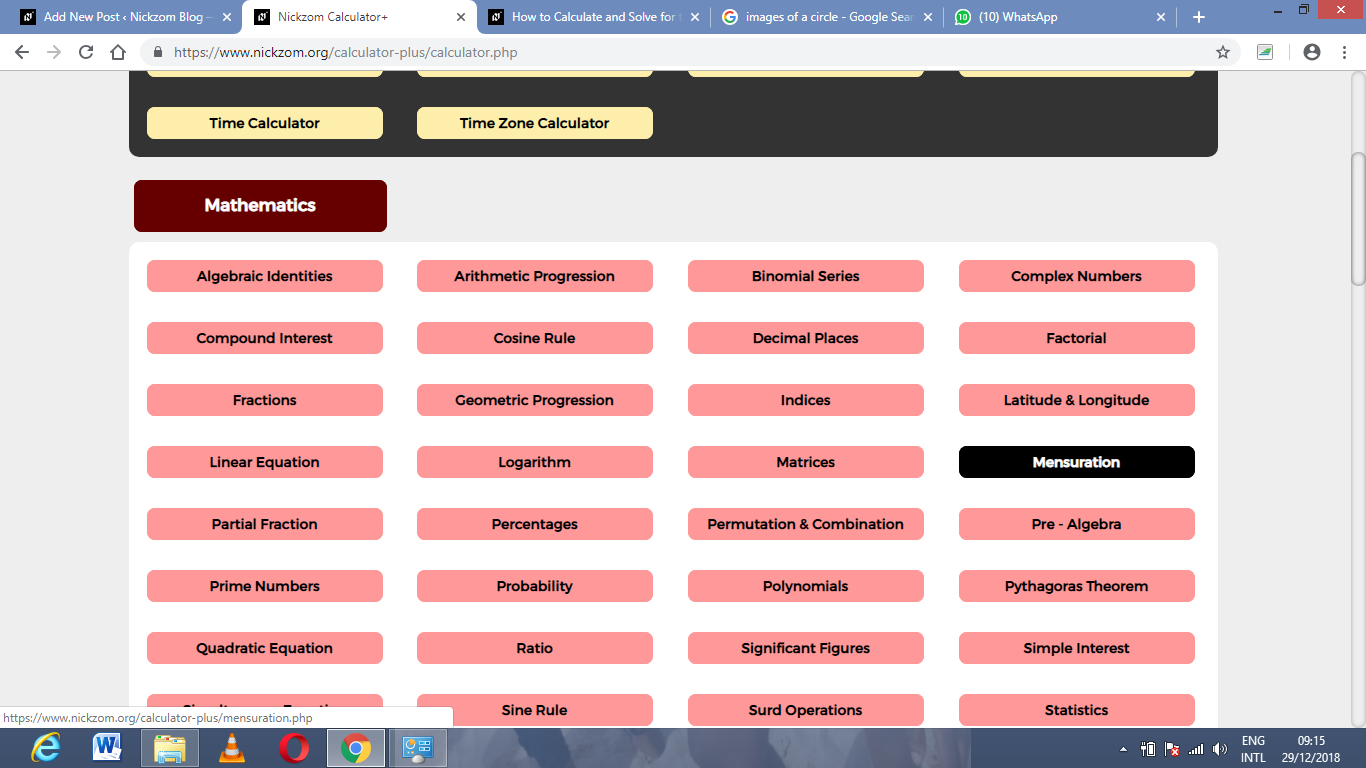Now, click on Area of a hexagon under Mensuration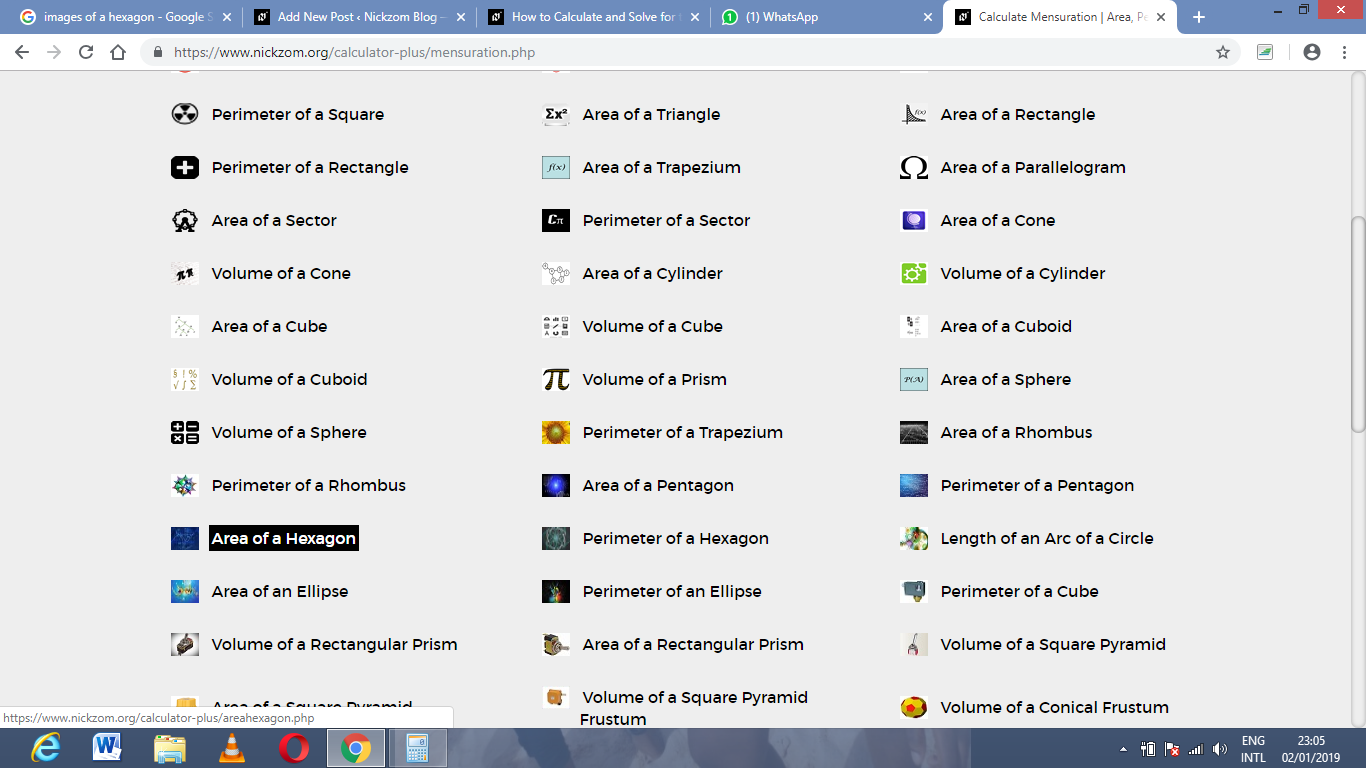The screenshot below displays the page or activity to enter your value, to get the answer for the area of a hexagon according to the respective parameter which is the length of side (a).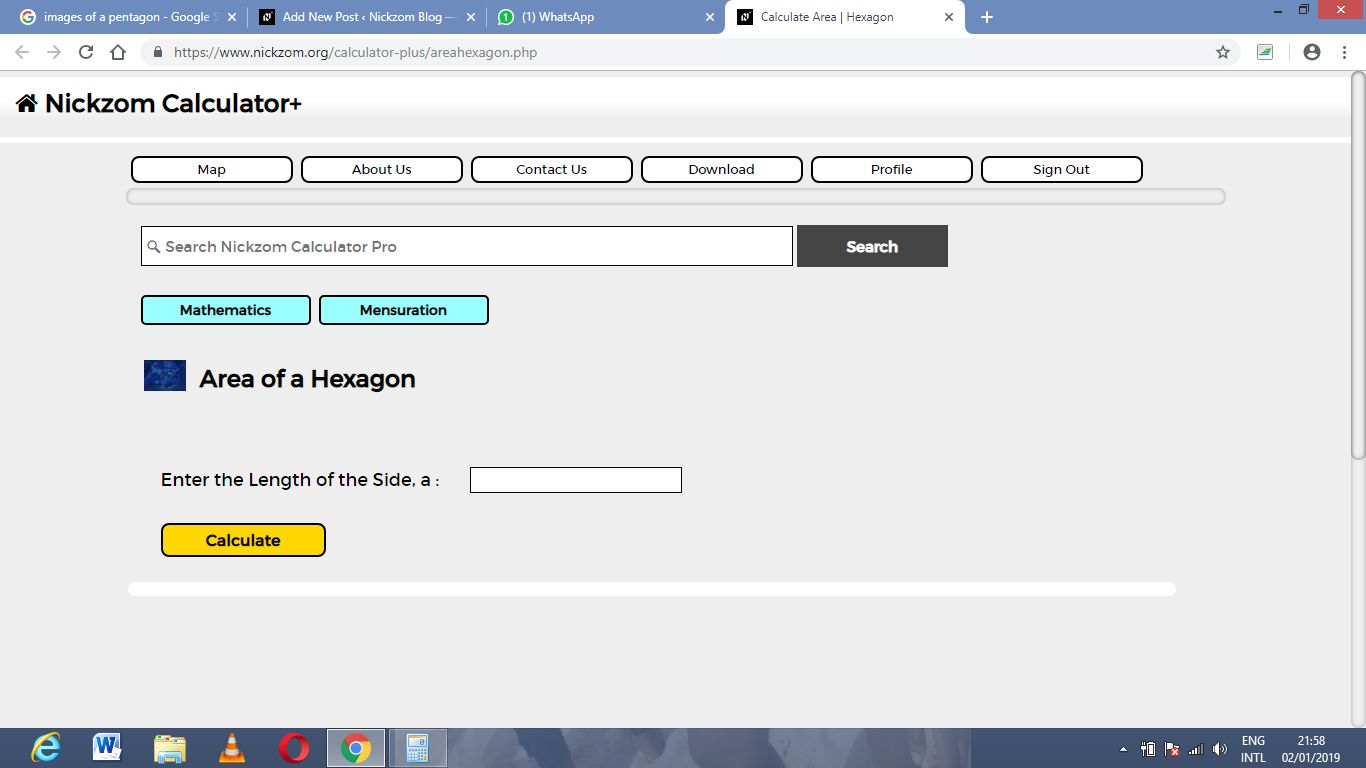Now, enter the values appropriately and accordingly for the parameter as required by the example above where the length of side (a) is 35 cm.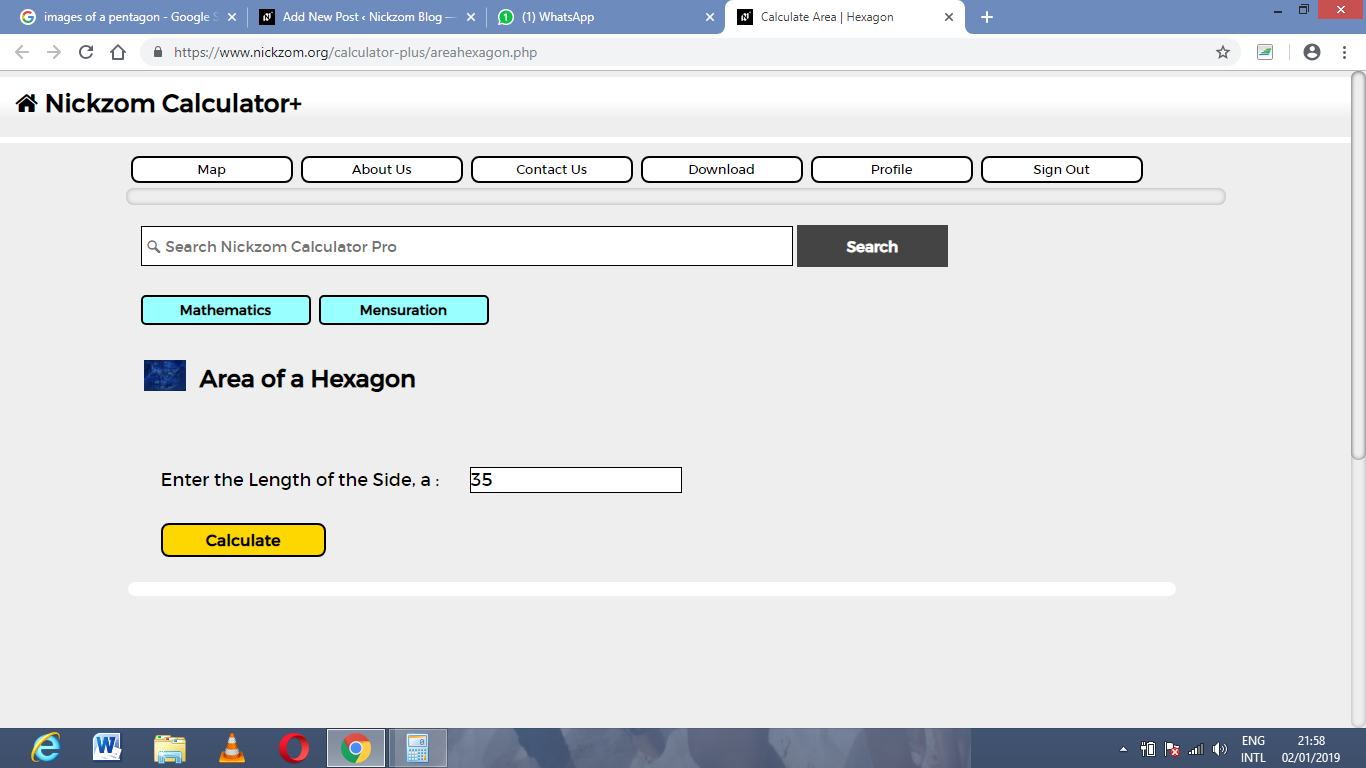Finally, Click on Calculate,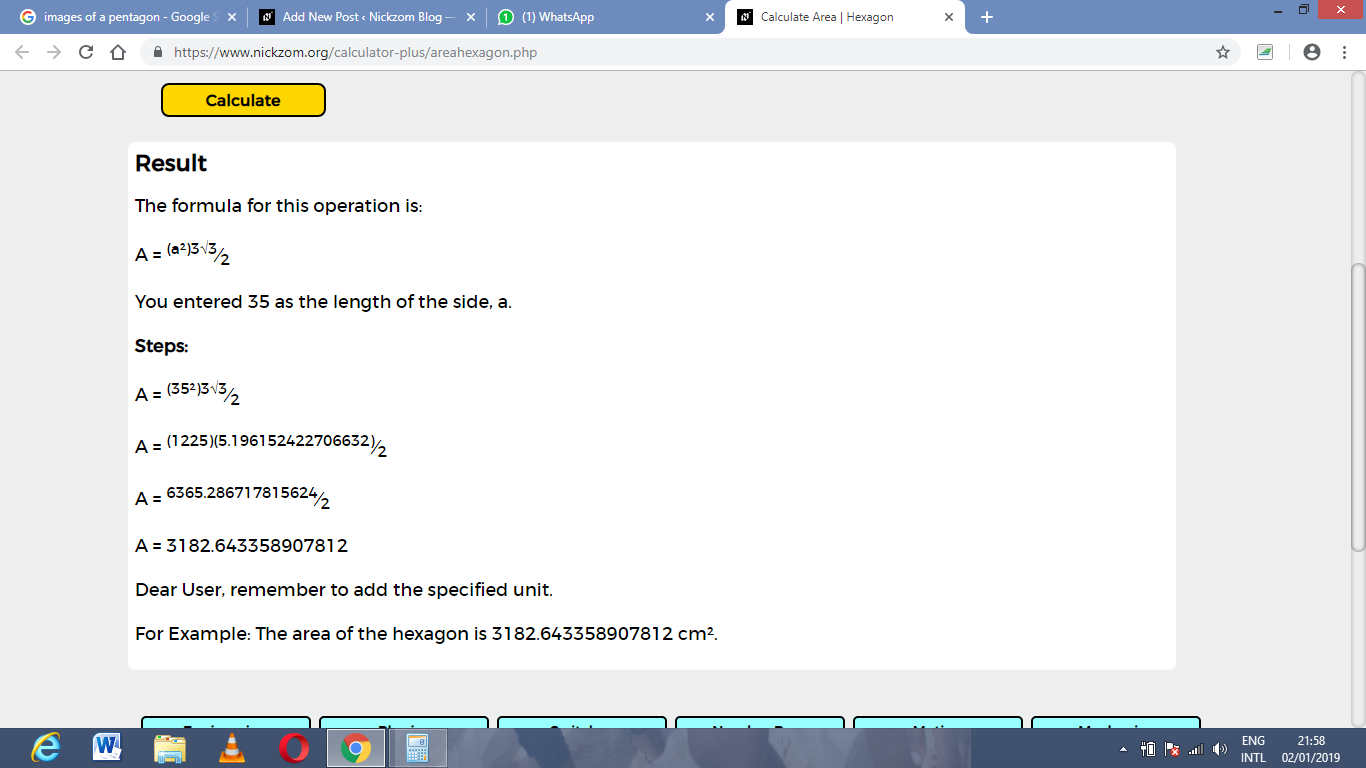As you can see from the screenshot above, Nickzom Calculator – The Calculator Encyclopedia solves for the area of a hexagon and presents the formula, workings and steps too.